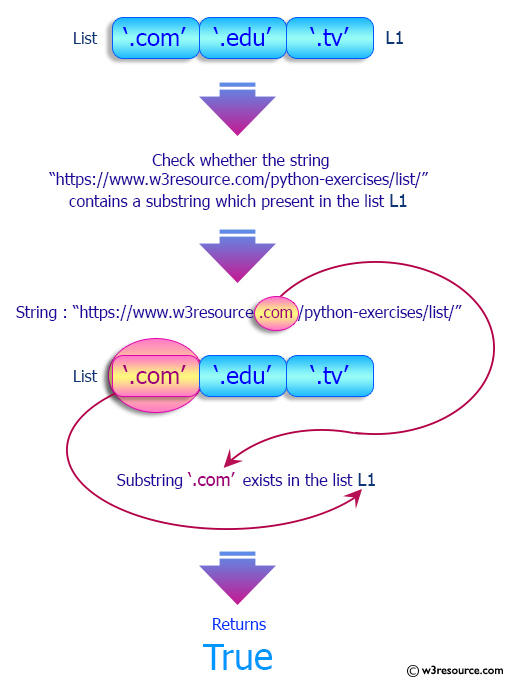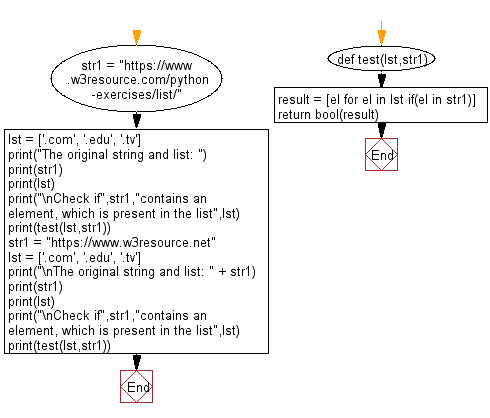﻿ Python: Check if a given string contains an element, which is present in a list - w3resource# Python: Check if a given string contains an element, which is present in a list

## Python List: Exercise - 201 with Solution

Write a Python program to check if a given string contains an element, which is present in a list.

Sample Solution:

Python Code:

``````def test(lst,str1):
result = [el for el in lst if(el in str1)]
return bool(result)

str1 = "https://www.w3resource.com/python-exercises/list/"
lst = ['.com', '.edu', '.tv']
print("The original string and list: ")
print(str1)
print(lst)
print("\nCheck if",str1,"contains an element, which is present in the list",lst)
print(test(lst,str1))
str1 = "https://www.w3resource.net"
lst = ['.com', '.edu', '.tv']
print("\nThe original string and list: " + str1)
print(str1)
print(lst)
print("\nCheck if",str1,"contains an element, which is present in the list",lst)
print(test(lst,str1))
```
```

Sample Output:

```The original string and list:
https://www.w3resource.com/python-exercises/list/
['.com', '.edu', '.tv']

Check if https://www.w3resource.com/python-exercises/list/ contains an element, which is present in the list ['.com', '.edu', '.tv']
True

The original string and list: https://www.w3resource.net
https://www.w3resource.net
['.com', '.edu', '.tv']

Check if https://www.w3resource.net contains an element, which is present in the list ['.com', '.edu', '.tv']
False
```

Pictorial Presentation:Flowchart:## Visualize Python code execution:

The following tool visualize what the computer is doing step-by-step as it executes the said program:

Python Code Editor:

Have another way to solve this solution? Contribute your code (and comments) through Disqus.

What is the difficulty level of this exercise?

Test your Python skills with w3resource's quiz

﻿

## Python: Tips of the Day

Floor Division:

When we speak of division we normally mean (/) float division operator, this will give a precise result in float format with decimals.

For a rounded integer result there is (//) floor division operator in Python. Floor division will only give integer results that are round numbers.

```print(1000 // 300)
print(1000 / 300)```

Output:

```3
3.3333333333333335```